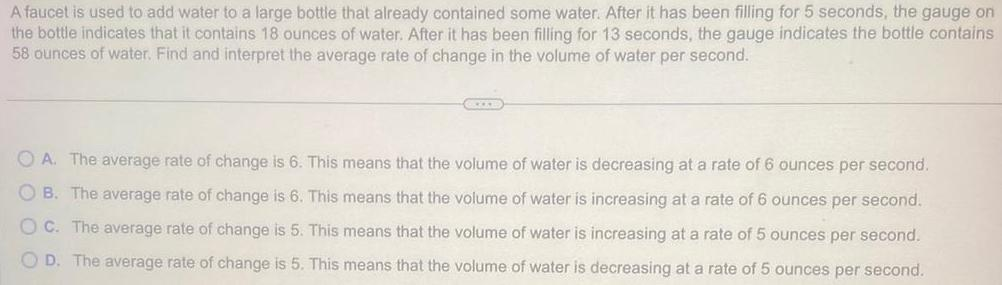Question:

# A faucet is used to add water to a large bottle that already

Last updated: 11/11/2023A faucet is used to add water to a large bottle that already contained some water After it has been filling for 5 seconds the gauge on the bottle indicates that it contains 18 ounces of water After it has been filling for 13 seconds the gauge indicates the bottle contains 58 ounces of water Find and interpret the average rate of change in the volume of water per second OA The average rate of change is 6 This means that the volume of water is decreasing at a rate of 6 ounces per second OB The average rate of change is 6 This means that the volume of water is increasing at a rate of 6 ounces per second OC The average rate of change is 5 This means that the volume of water is increasing at a rate of 5 ounces per second OD The average rate of change is 5 This means that the volume of water is decreasing at a rate of 5 ounces per second